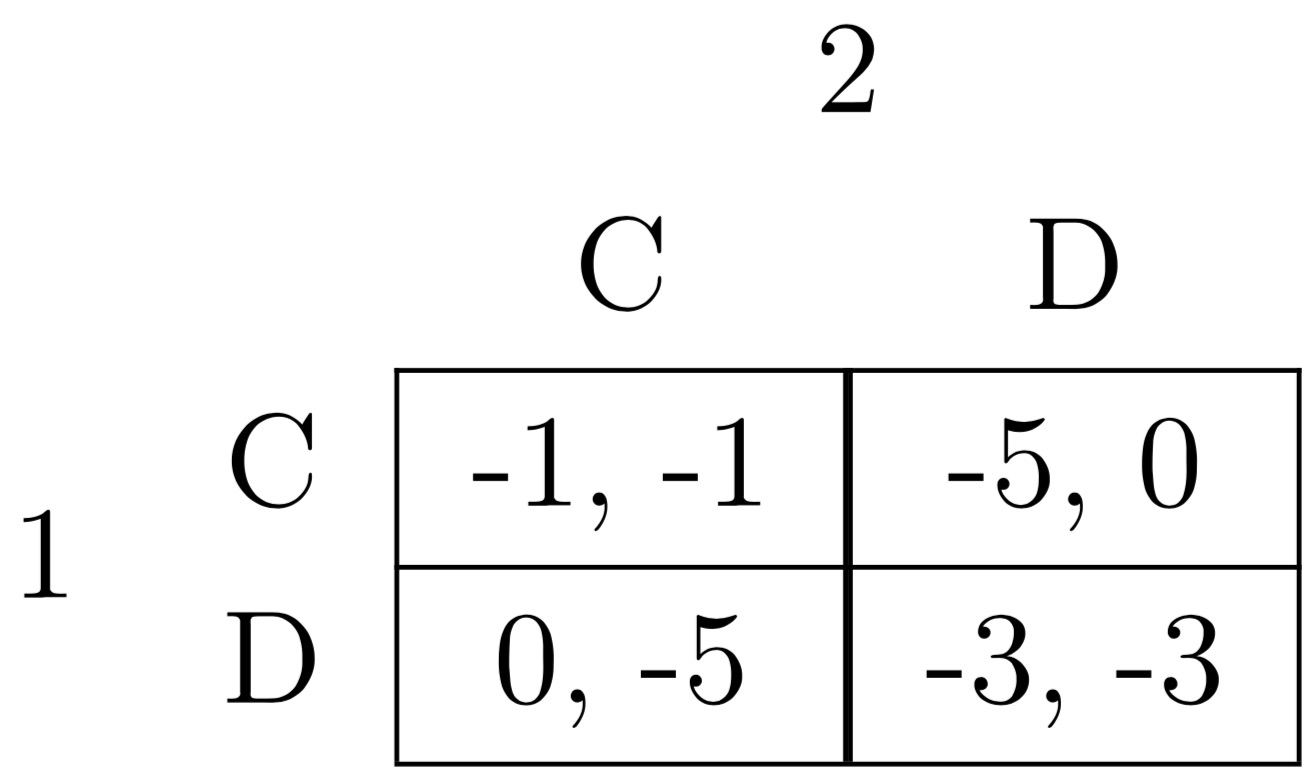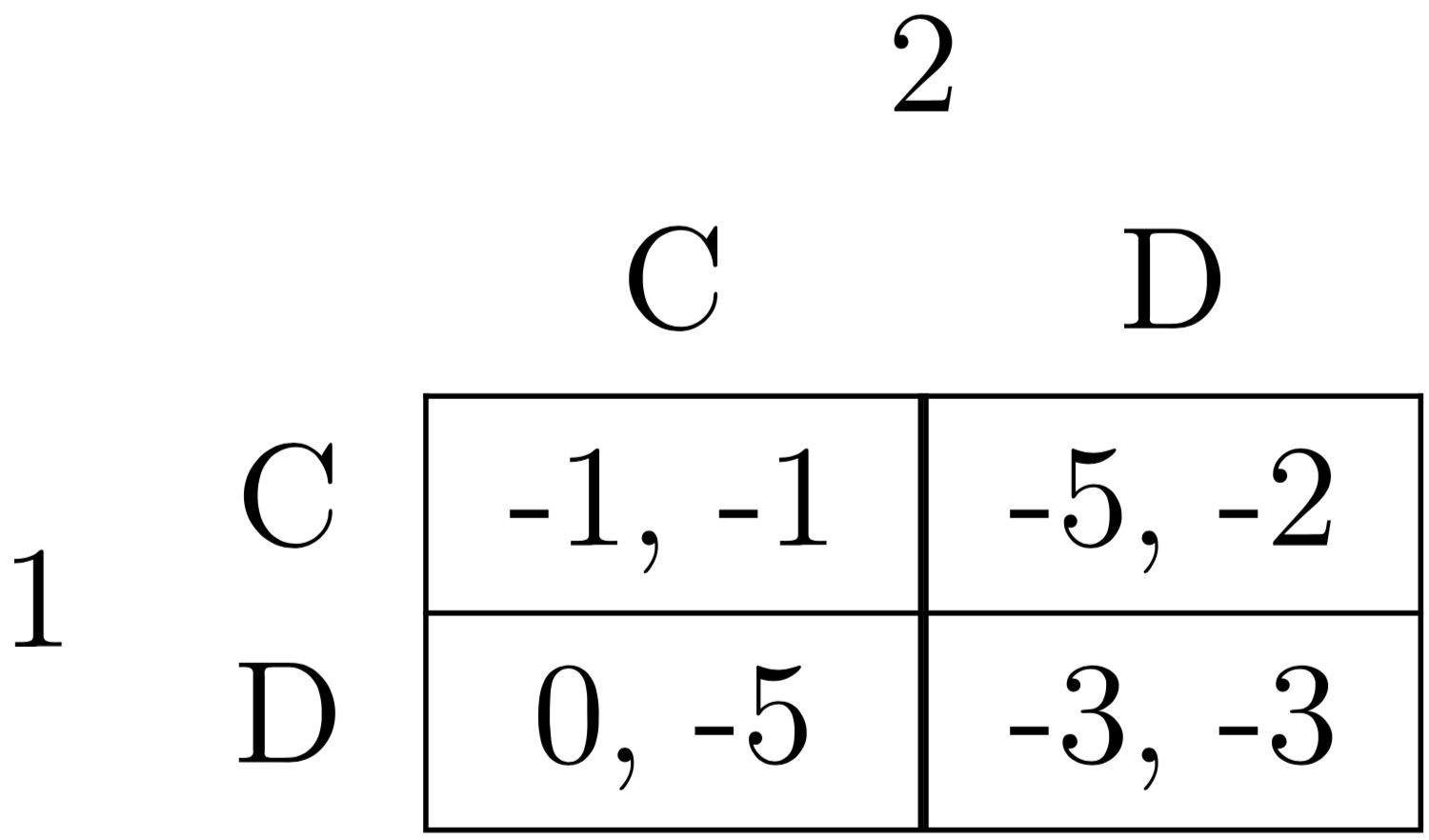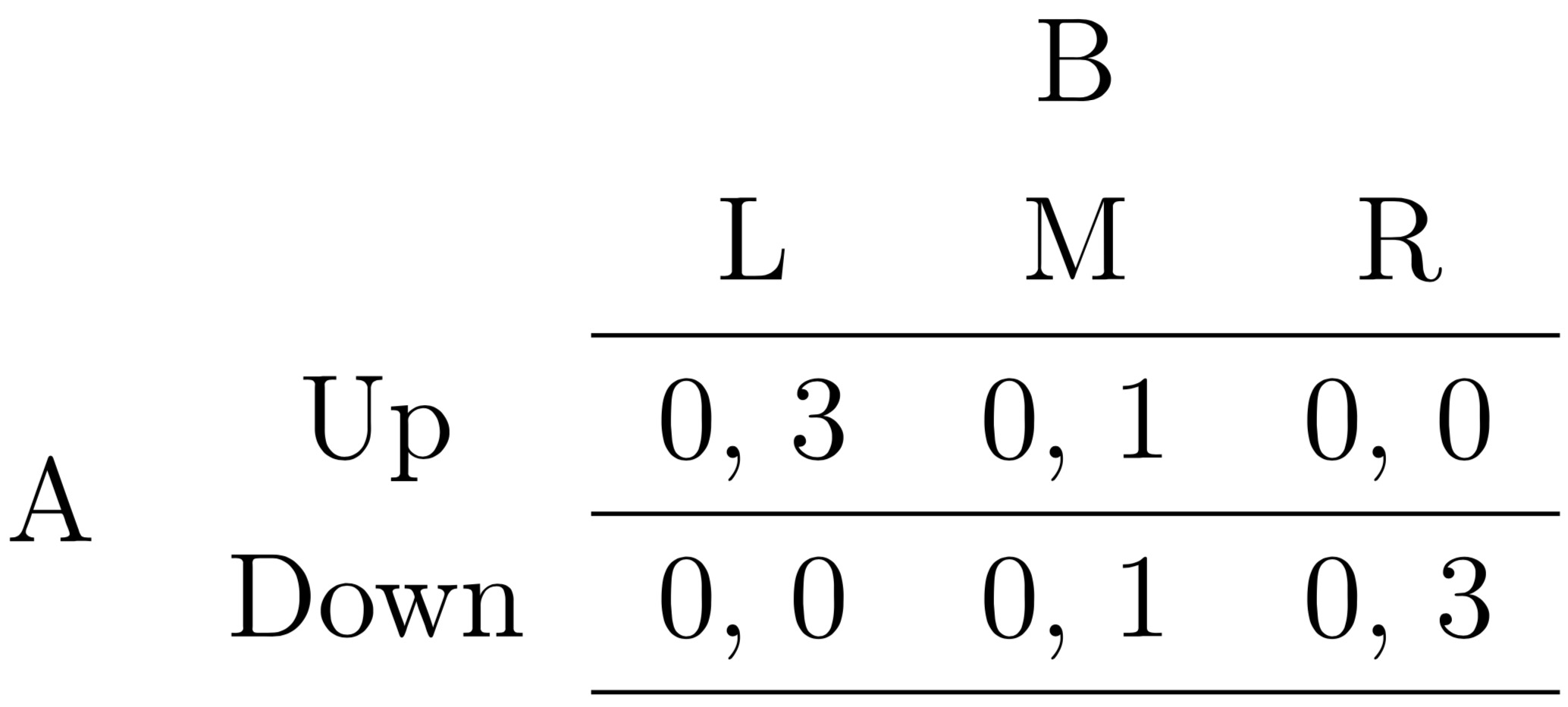## Game Theory Basics

### Definition: Normal Form Game

• Players $i \in 1..n$
• For every player $i$, $\exists$ a set of strategies $S_i : s_i \in S_i$
• $\forall i \land \forall S_i, S = \{s_1..s_n\} \lor S = \{s_i,S_j\}: s_j = \{s_1 .. s_{i-1}, s_{i+1} .. s_n \}$

### Notation

If there are two players, $i \land j \implies U_i(s_i,s_j) \land U_j(s_j,s_i)$. Note that discussions of utility and strategy choices always take the player in perspective as the first argument and the other players are subsequent arguments.

## Prisoner’s Dilemma

$\exists i \in {1,2}:$Suppose that you are Player 1. You will make the following call:

If $S_2=C$, then you will have: $\begin{cases} U_1(C,C) &= -1 \\ U_2(D,C) &= 0\end{cases} \implies S_1=D$

If $S_2=D$, then you will have $\begin{cases} U_1(C,D) &= -5 \\ U_2(D,D) &= -3\end{cases} \implies S_1=D$

If you suppose that 1 thinks that $P(S_2=C) = q$ and $P(S_2=D)=1-q$, we have that:

$$\begin{cases} U_1(C,q) = q(-1) + (1-q)(-5) &= 4q-5 \\ U_2(D,q) = q(0) + (1-q)(-3) &= 3q-3 \end{cases}$$

Since $3q-3 > 4q-5 \ \forall \ q \in [0,1]$, 1 should defect. However, there is a better choice if they work together. But, if both are rational and only self interested, they will end in a worse position. In this situation, we say that $D$ strictly dominates $C$ and $C$ is strictly dominated by $D$.

### Remark: Importance

We can see that for both players, their dominant strategy would be (D,D). However, this strategy is Pareto dominated by another strategy, (C,C). This is a direct counterexample to the previously Adam Smith’s First Welfare Theorem which suggested that com- petitive markets intrinsically tended to the efficient allocation of resources. However, this is not the case in the Prisoner’s dilemma, where the efficient allocation (Pareto dominant) would be (C,C)

However, we can solve the prisoner’s dilemma through a few possible solutions:

• Binding contracts between parties with third party enforcement (e.g. courts)
• Repeated Games

### Different Utilities

Suppose there is a modified prisoner’s dilemma as follows:Consider the modification to Player 2’s defect scenario as a cost of defecting. Note that for Player 1, nothing changes and defecting will still be his best strategy. However, we adjust Player’s 2 perspective as follows:

$$S_1 = D \implies \begin{cases} U_2(C,D) &= -5 \\ U_2(D,D) &= -3 \end{cases} \implies S_2=D$$

$$S_1 = C \implies \begin{cases} U_2(C,C) &= -1 \\ U_2(D,C) &= -2 \end{cases} \implies S_2=C$$

Suppose that $P(S_1=C) = p$. Therefore, we have:

$$\begin{cases} U_2(C,p) = p(-1) + (1-p)(-5) &= 4p-5 \\ U_2(D,p) = p(-2) + (1-p)(-3) &= p-3 \end{cases} \Rightarrow 4p-5 > p-3 \rightarrow 3p>2 \implies$$

$$\begin{cases} p>\frac{2}{3} \implies S_2=C \\ p<\frac{2}{3} \implies S_2=D \end{cases}$$

## Definitions

### Definition: Strictly Dominates

A strategy $S_i$ strictly dominats $S_i’$ if $\forall S_j \rightarrow U_i(S_i,S_j) > U_i(S_i’,S_j)$. In other words, $S_i$ strictly dominates if it is strictly better no matter what the other player does. We can also say that $S_i’$ is strictly dominated by $S_i$.

### Definition: Strictly Dominant

If $S_i$ strictly dominates all other $S_i’$, then $S_i$ is strictly dominant. Note that even if tehre are strategies that strictly dominate another one, that does not mean that there must be a strictly dominant strategy.

Note that you can attempt to identify strictly dominant strategies and eliminate those options, since they are “bad” ways of playing the game. Instead, you can also attempt to find “good” ways of playing the game.

### Definition: Expected Value

Let $X$ be a random variable that takes vlaues $X_i$ with probability $p_i: i \in \{1..n\}$. The expected value of $X$ is defined as:

$$E[X] = p_1x_1 + … + p_nx_n$$

### Definition: Belief of Player

A belief of a player $i$ is a probability distribution $\sigma_j \in \Delta(S_j)$ that assigns probability $\sigma_j(S_j)$ to a strategy profile $S_i$. Essentially, you are assigning a weight to the chance of you playing a certain strategy. Given the beliefs, expected utility from $S_i$ given $\sigma_j$ is defined as:

$$U_i(S_i,\sigma_j) = \sum_{s_j}{u_i(s_i,s_j) \cdot \sigma_j(s_j)}$$

### Best Response

A strategy $s_i$ is a best response to beliefs $\sigma_j$ if it maximizes $E[U_i]$ such that:

$$U_i(s_i,\sigma_j) \ge U_i(s_i’,\sigma_j) \ \forall s_i’$$

If this condition is satisfied, $BR_i(\sigma_j)$ is the set of best responses to $\sigma_j$.

### Example: Modified Prisoner’s Dilemma

Taking another look at our modified Prisoner’s Dilemma from earlier:Let $\sigma_j =: \sigma_j(C) = p$. Therefore

$$U_2(C,\sigma_j) = 4p-5$$ $$U_2(D,\sigma_j) = p-3$$

Therefore, we have the following best response:

$$BR_2(\sigma_j) \begin{cases} C & p>\frac{2}{3} \\ D & p<\frac{2}{3} \\ [C,D] & p=\frac{2}{3} \end{cases}$$

## Relationship between Dominance and Best Response

### Question

Is there a relationship between strictly dominated strategies and best responses to a set of beliefs?

### Theorem

• If $S_i’$ is strictly dominated by some $S_i$, then it can not a best response to any set of beliefs $\sigma_j$.

#### Proof

Pick any $\sigma_j:$

$$U_i(S_i,\sigma_j) = \sum_{s_j}{u_i(s_i,s_j) \cdot \sigma_j(s_j)}$$

Note that $u_i(s_i,s_j) > u_i(s_i’,s_j)$ since $s_i$ strictly dominates $s_i’$. Therefore:

$$U_i(s_i,\sigma_j) > U_i(s_i’,\sigma_j)$$

Therefore $s_i’$ is not a best response to $\sigma_j$

### Question

If $s_i’$ is never a best response, it is strictly dominated.

This question is difficult to answer but an answer can be shown in the following example.

### Example

Suppose the following game:for $\sigma_A: \sigma_A(U) =p$.

$$U_B(S_B,\sigma_A) = \begin{cases} 3p & S_B=L \\ 1 & S_B = M \\ 3(1-p) & S_B=R \end{cases}$$

We see that $M$ is never considered a best response. However, M is never strictly dominated in this game. Consider a mixed strategy by player B chosen by a coin flip s.t.:

$$\begin{cases} L & \text{if heads} \\ R & \text{if tails} \end{cases}$$

This strategy gives 1.5 no matter that $A$ will end up playing. This strategy strictly dominates $M$.

### Theorem

If $S_i$ is not a best response, then it is strictly dominated [possibly] by a mixed strategy.